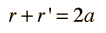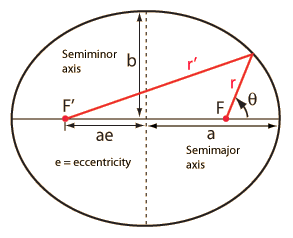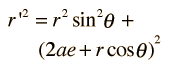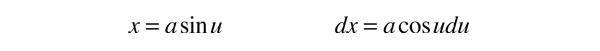# Ellipses and Elliptic OrbitsAn ellipse is defined as the set of points that satisfies the equationIn cartesian coordinates with the x-axis horizontal, the ellipse equation isThe ellipse may be seen to be a conic section, a curve obtained by slicing a circular cone. A slice perpendicular to the axis gives the special case of a circle.

For the description of an elliptic orbit, it is convenient to express the orbital position in polar coordinates, using the angle θ:Show
This form makes it convenient to determine the aphelion and perihelion of an elliptic orbit. The area of an ellipse is given byShow
 Each of the conic sections can be described in terms of a semimajor axis a and an eccentricity e. Representative values for these parameters are shown along with the types of orbits which are associated with them.Index

 HyperPhysics*****HyperMath*****Geometry R Nave
Go Back

# Polar Form of EllipseFrom the diagram at left, using the Pythagorean theorem to express r' in terms of r:Using the trigonometric identitythis reduces toUsing the equation for an ellipse, an expression for r can be obtainedThis form is useful in the application of Kepler's Law of Orbits for binary orbits under the influence of gravity.

Index

Orbit concepts

Carroll & Ostlie
Sec 2.1

 HyperPhysics*****HyperMath*****Geometry R Nave
Go Back

# Area of EllipseUsing the equation for an ellipsethe height y can be expressed asand integrated over a quarter of the ellipse to get the area:This kind of integral may be evaluated by using trigonometric substitutionThis gives the area integralUsing the trigonometric identitythe integral becomesThen using the trigonometric identitythis givesIndex

Orbit concepts

Carroll & Ostlie
Sec 2.1

 HyperPhysics*****HyperMath*****Geometry R Nave
Go Back